# 基于参数化模型(MANO)的手势姿态估计---全面剖析

### 1. 什么是手部姿态估计？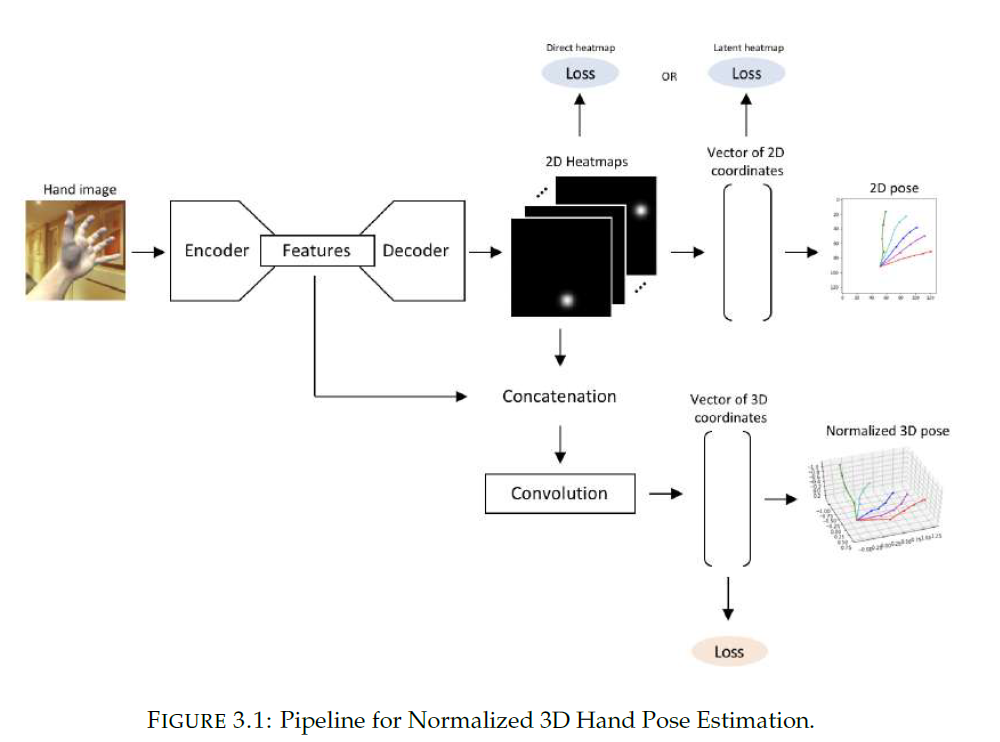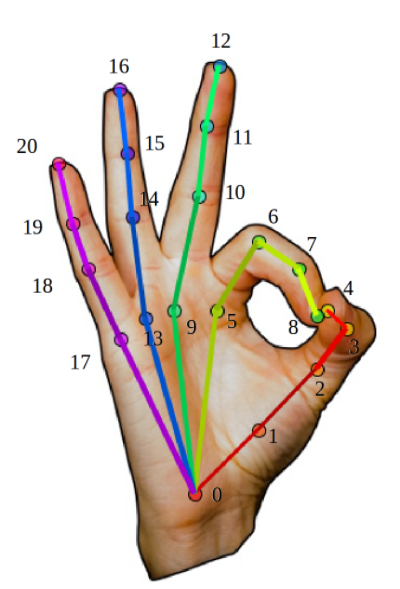img = cv2.imread("xxx.jpg")  # 1) 读取手部特写图片
img = normalize(img)         # 2) 对图片进行处理(规则化等)
pred_3d = Net(img)           # 3) 送入网络进行预测
pred_3d -= pred_3d        # 4) 将预测结果变为root-relative的方式.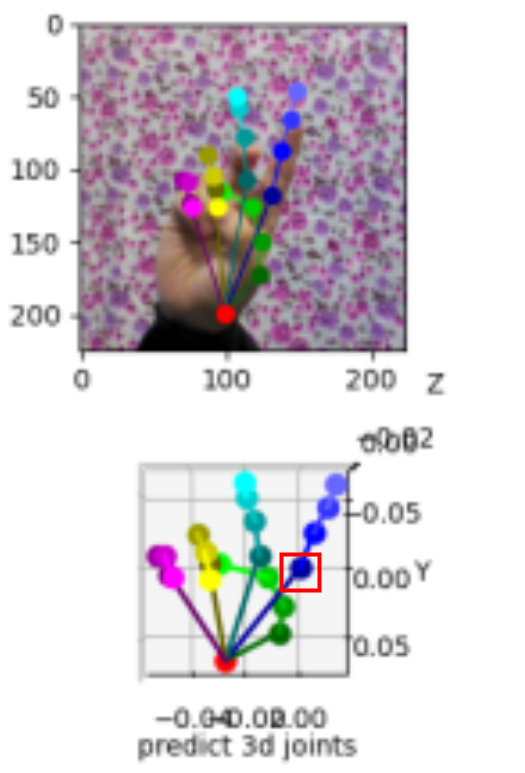### 2. MANO是什么？

To cope with low-resolution, occlusion, and noise, we develop a
new model called MANO (hand Model with Articulated and Non-rigid defOrmations).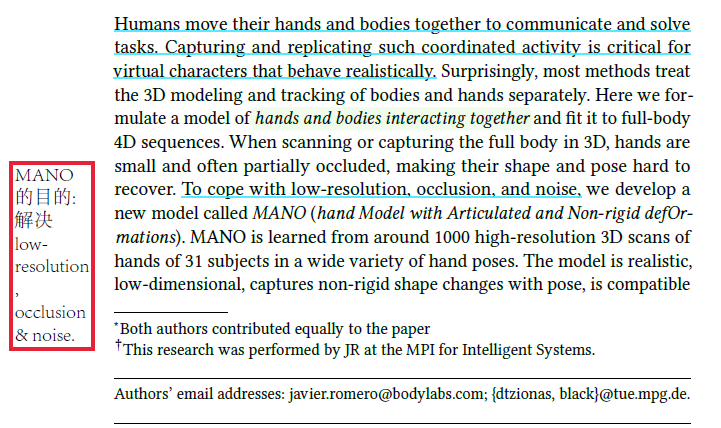图来自.

• 自遮挡: 以下图为例，中指到小指都被手背挡住了.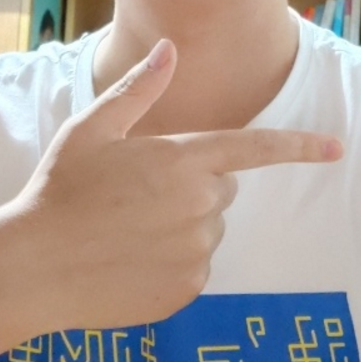• 手抓握物体导致的遮挡: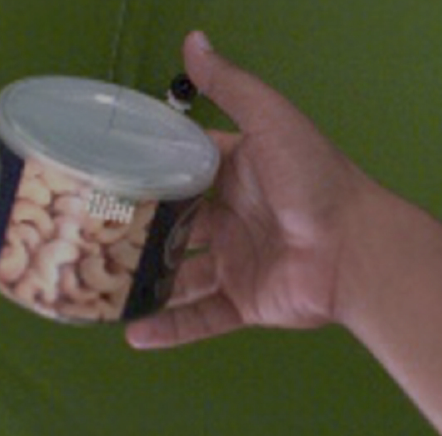• 分辨率低: 手部在整个构图中的所占像素比例非常小，对正确估计其手势增加了难度.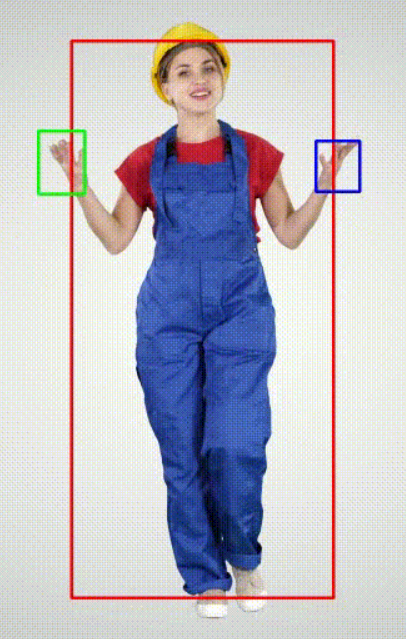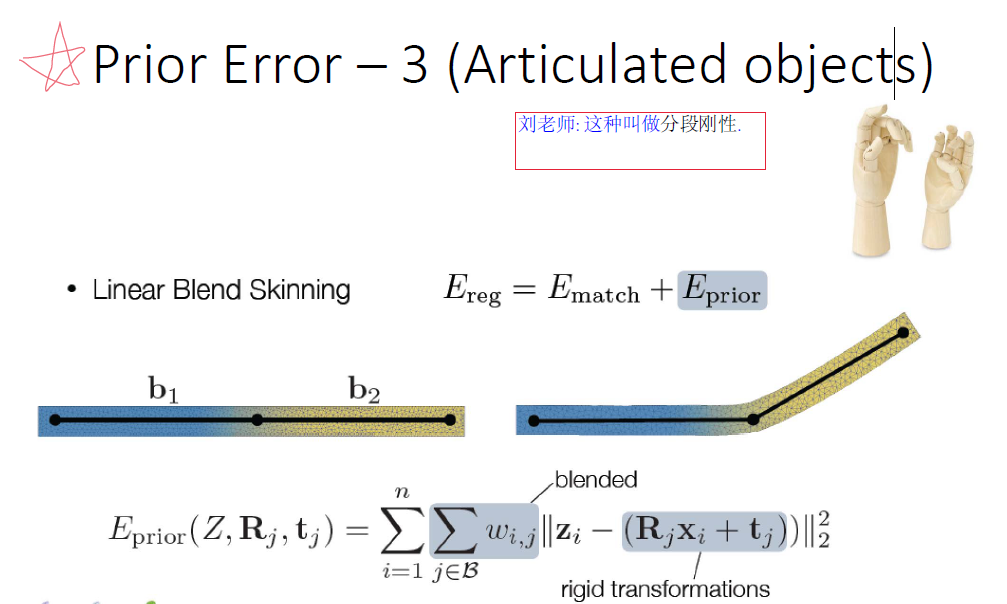• 778个vertices 1538个faces，并根据 16个 关键点 +从顶点中获取 5个
手指指尖的点， 构成完整的手部链条，或者叫做前向动力学树 (forward kinematic tree).
下图来自牛津大学的CVPR2019论文《3D Hand Shape and Pose from Images in the Wild》.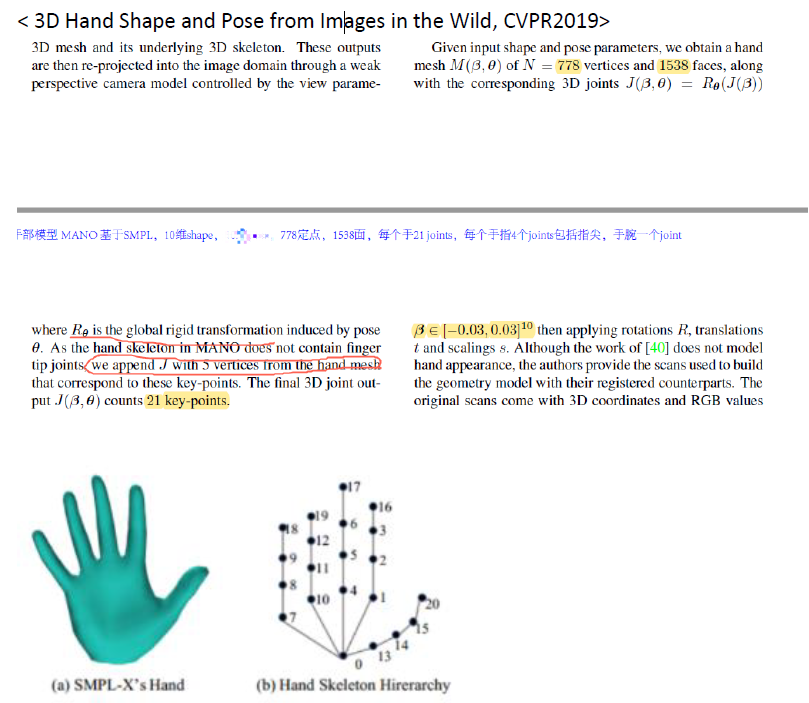(即图中的 ,16,17,18,19,20都是由变形后的 vertex中按照规定规则取到的 , 代码. 以节点9为例，其3D坐标可以在变形后的Mesh上，根据其所在顶点(vertex)去提取。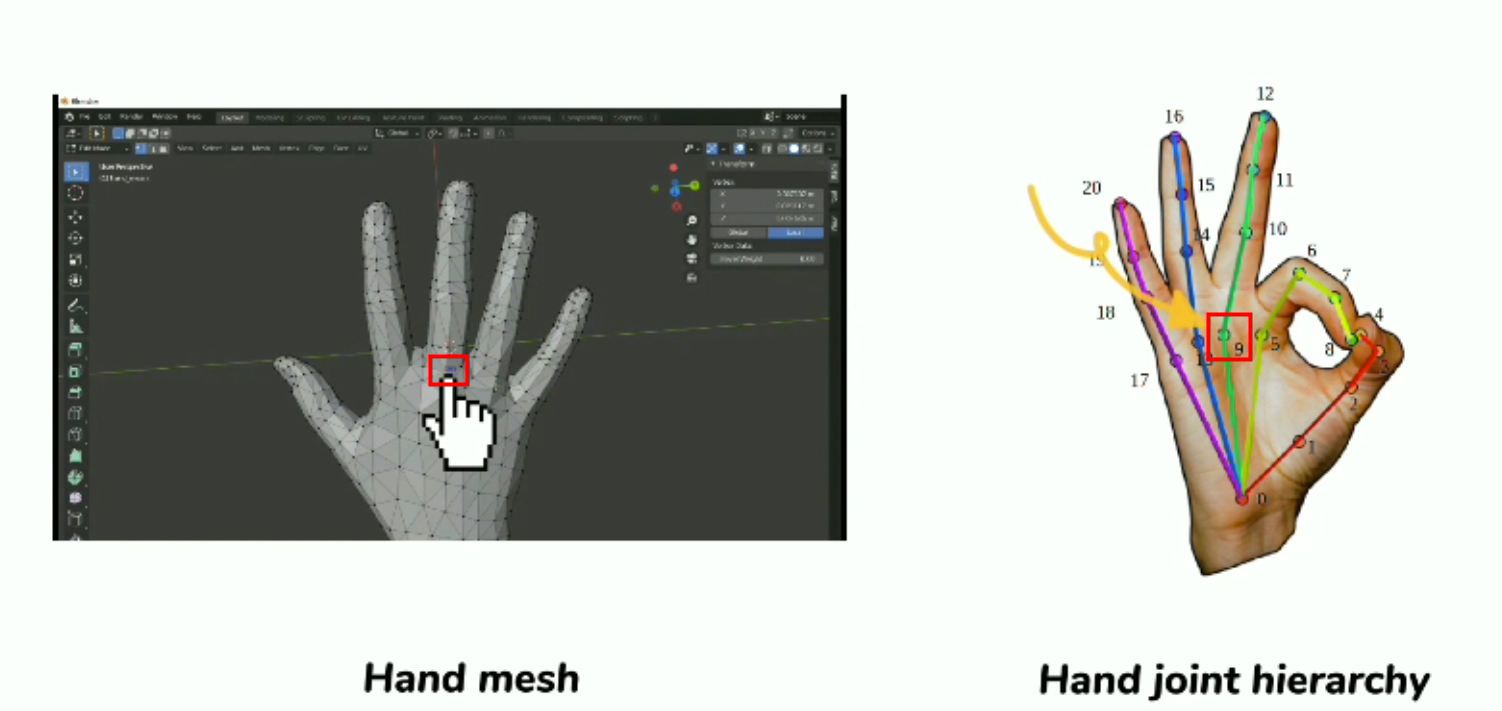### 3. 手势3D pose estimation的MANO部分处理逻辑分析重点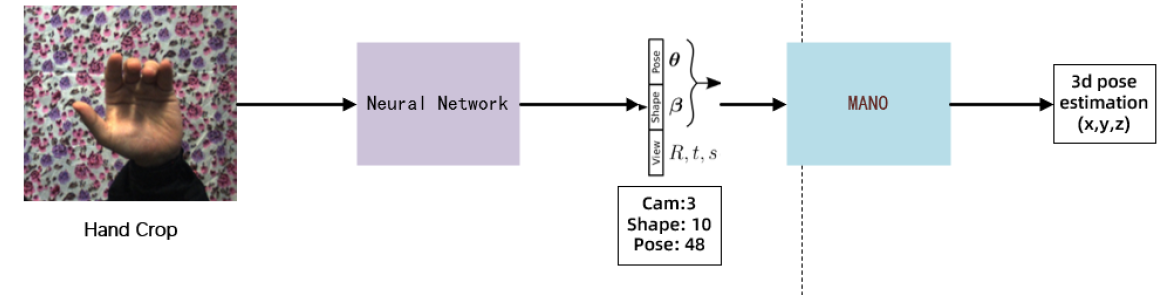• 输入(bs,3,224,224)
为图像处理后得到的Tensor(NCHW，图像的分辨率可以按照自己的需求调整), bs是batch size.

• 输出为(bs, 61)
得到MANO所需的参数, 这些参数输入MANO, 我们就可以得到3D的pose estimation结果(相对坐标系下的21个关键点的xyz位置).

#### 3.1 MANO的计算逻辑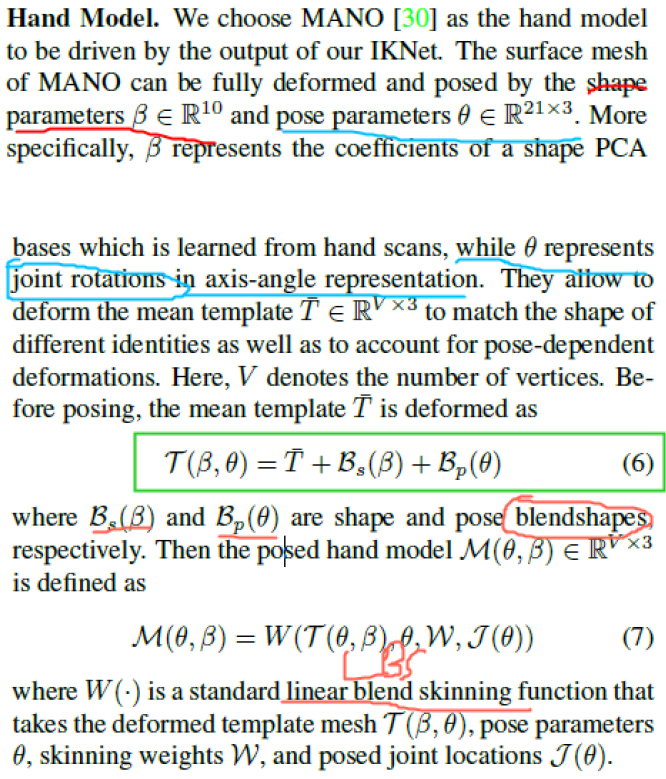• T ( β , θ ) = T ‾ + B s ( β ) + B p ( θ ) T(\beta, \theta) = \overline{T} + B_s(\beta) + B_p(\theta)
此公式是将shape的参数 β ∈ R 10 \beta \in R^{10} 和pose的参数 θ ∈ R 48 \theta \in R^{48} 进行变形，变形的实现通过 B s / p B_{s/p} 实现。 T ‾ \overline{T} 表示一个标准的3D 手部mesh, 下图就是MANO的hand_mean T ‾ \overline{T} :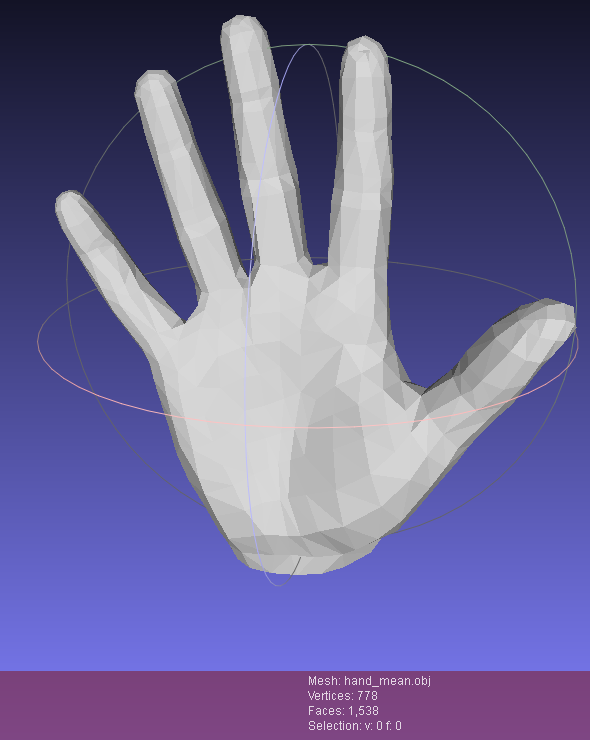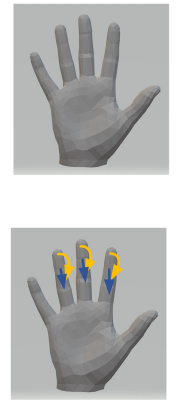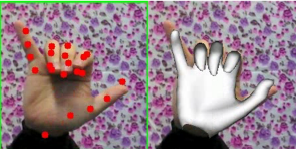• M ( β , θ ) = W ( T ( θ , β ) , θ , β , W , J ( θ ) ) M(\beta, \theta) = \mathbf{W}(T(\theta, \beta), \theta, \beta, W, J(\theta))
通过第①步，我们得到变形后的mesh: T ( θ , β ) T(\theta, \beta) , 第②步的目的就是进行蒙皮操作(linear blend skinning), W W 蒙皮权重 J ( θ ) J(\theta) 节点的位置.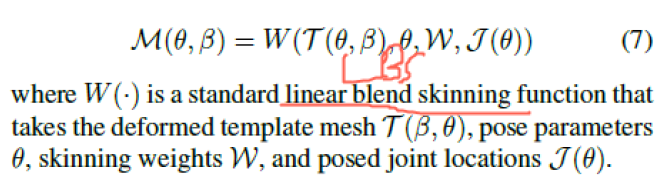#### 3.2 MANO的实际计算流程

• rots ∈ R 3 \in R^{3}
root节点(手腕CMC)的旋转axis-angle.
• poses ∈ R 45 \in R^{45}
除每个手指指尖外(除TIP这一排)和手腕外，所有的关键点的axis-angle (15*3=45), 下图来自ECCV2020 BMC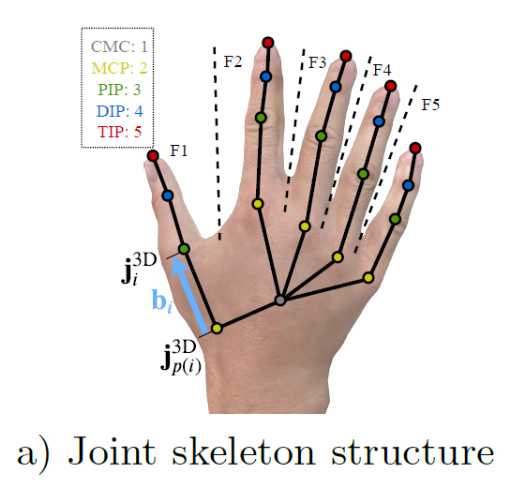• betas ∈ R 10 \in R^{10}
mano所需要的shape参数
##### 3.2.1 MANO的一些参与计算的重要参数
• kintree_table, parent 等动力学与继承关系参数
如下图所示，parent和 kintree_table组建了手势的链条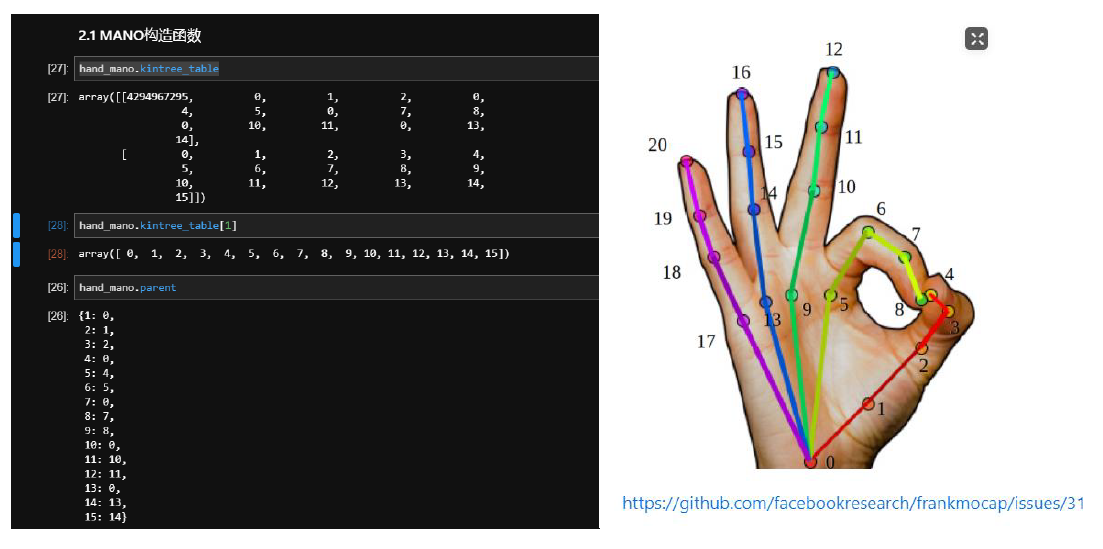注意，kintree_table是 不包含指尖 的 ！！(只有 16个 joints 15个手指的 joints+1个 wrist joint)，也符合 MANO定义的结构形式，即没有上图的(4,8,12,16,20)这5个点.

• hands_mean: 和 mesh_mu类似，这里的 hands_mean应该
是rest的手的axis-angle， 其用法是用来加上网络预测的 pose (axis-angle)，再对其进行处理。
需要注意的是，这里的pose除了不包含TIP骨骼外，还不包括手腕.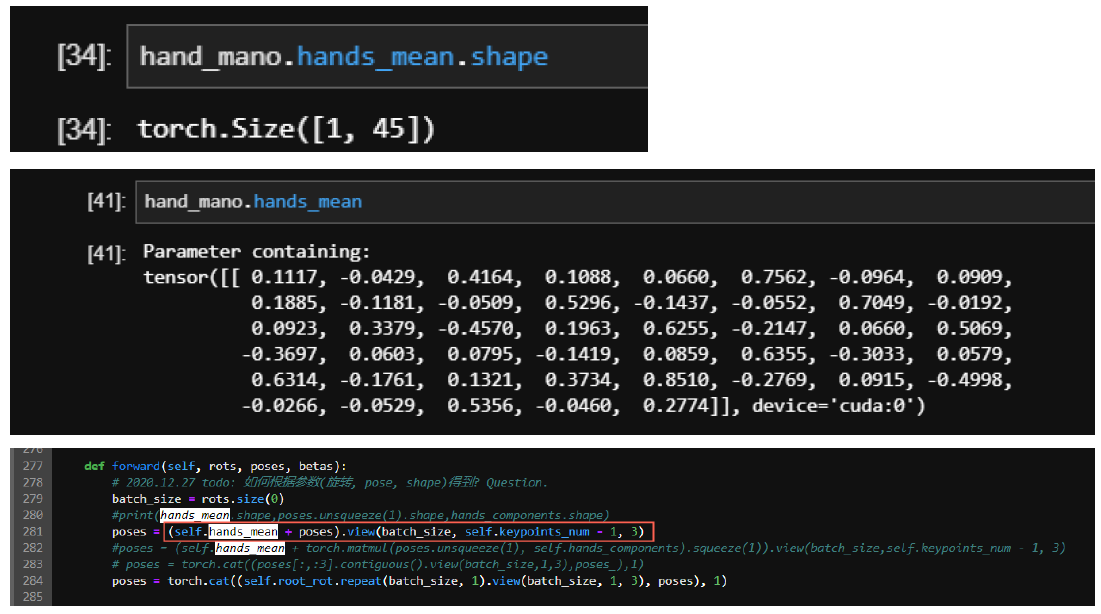• mesh_mu: 下图 (MANO2017)的公式 2的 T ‾ \overline{T} . 即平均 shape。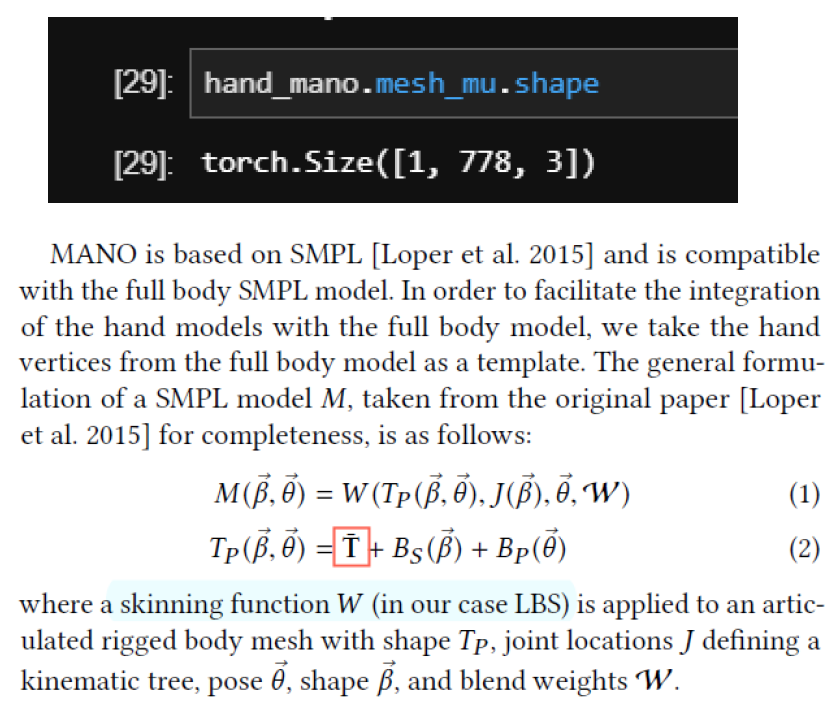公式2的计算得到的 T p T_p 对应代码中的 v p o s e d v_{posed} :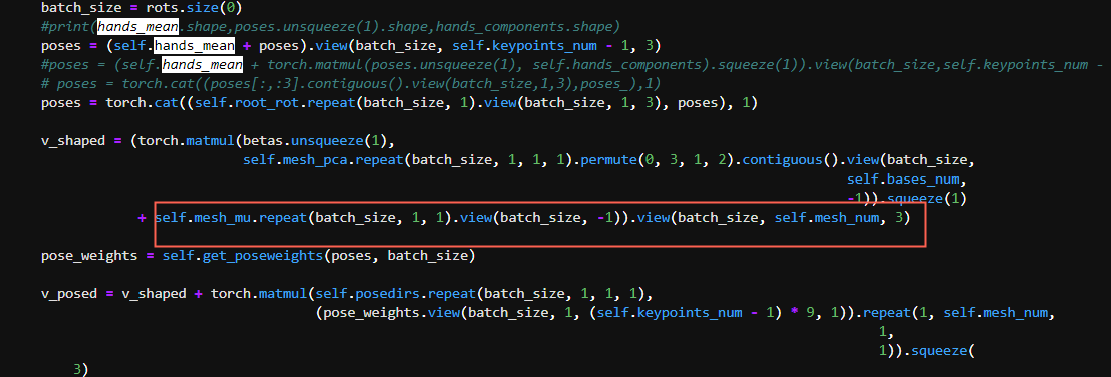• mesh_pca: 对应最大的 shape特征值的特征向量 , 因为 shape是根据pca取最大的 10维参数作为 shape的，这里的 mesh_pca根据其用法，是公式 4(MANO2017)里面的Sn。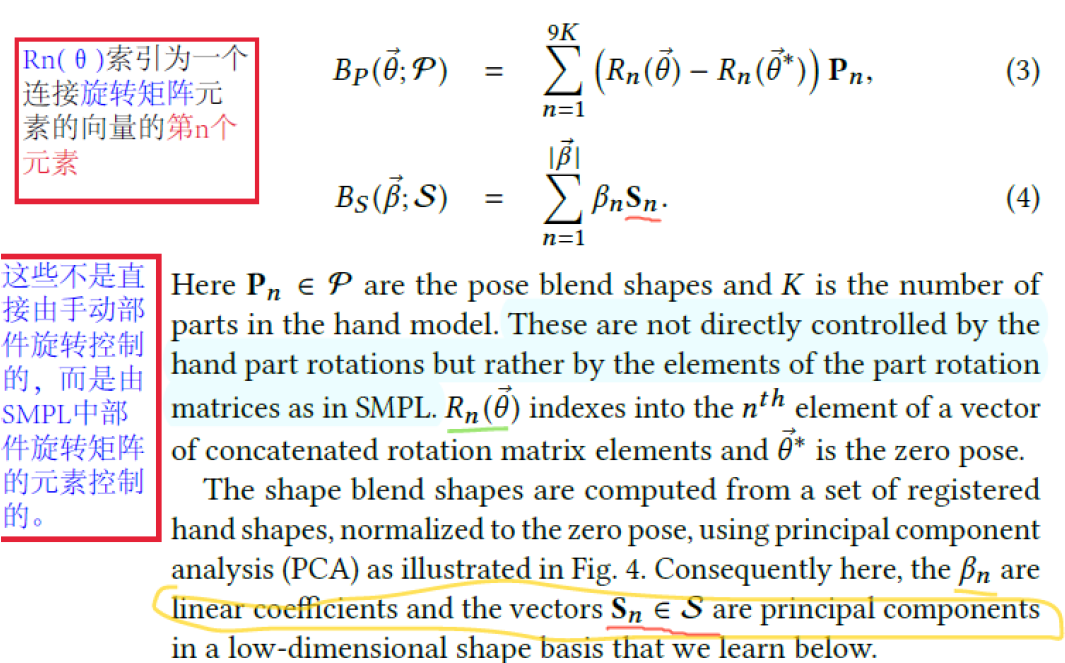对应代码: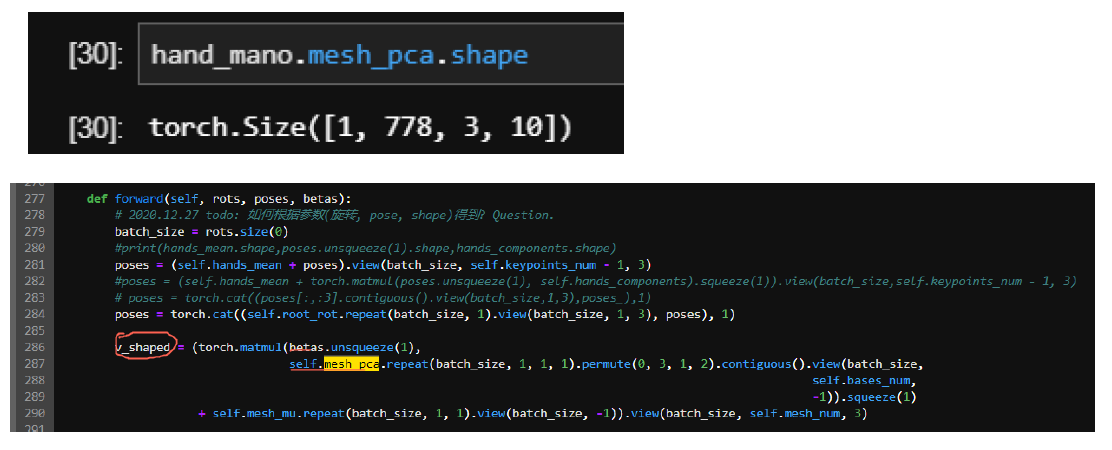• J_regressor: 对应 (SMPL2015)的公式10, 其目的是: 将mesh上的顶点 (vertices)变为节点 (joints).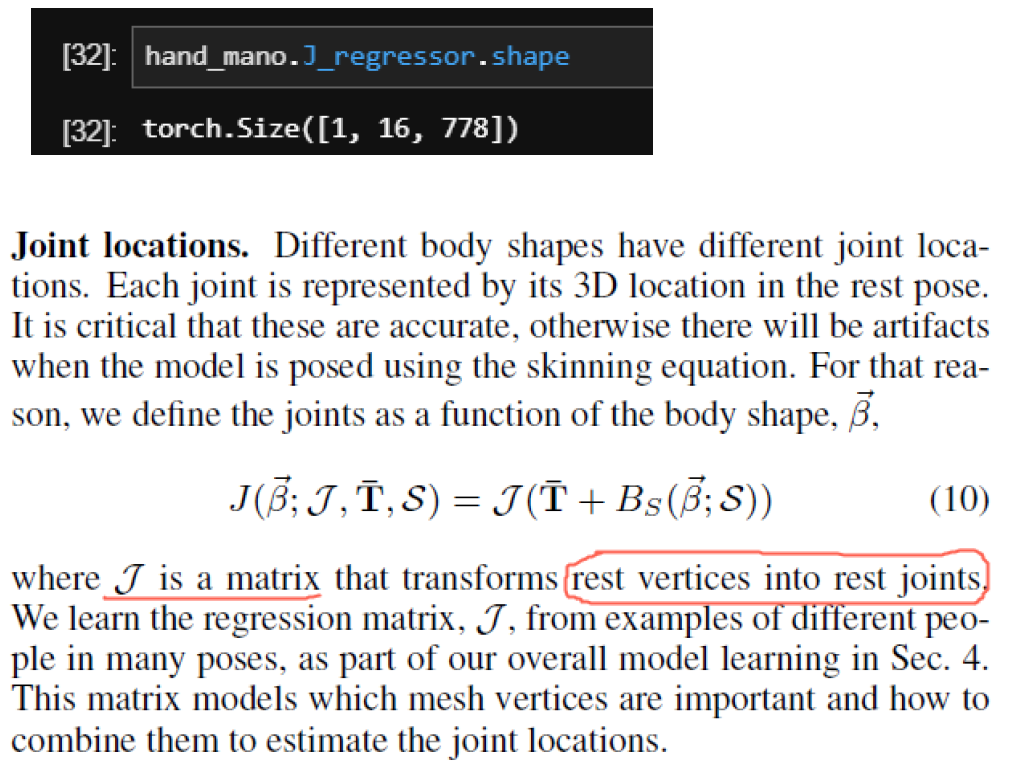• root_rot: 根节点, 是指手腕的那个点 即下图的 0点 ，我理解这里应该是用这种方式来计算相对的距离。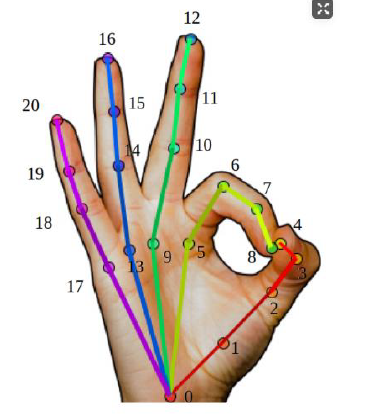• posedirs: 根据之前的分析， posedirs就是MANO(2017)中公式3中的Pn, 表示pose的blend shape参数.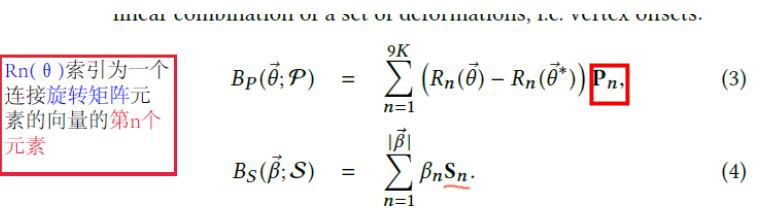• weights: weights就是3.1中公式2 M ( β , θ ) = W ( T ( θ , β ) , θ , β , W , J ( θ ) ) M(\beta, \theta) = \mathbf{W}(T(\theta, \beta), \theta, \beta, W, J(\theta)) W W .

##### 3.2.2 旋转矩阵计算(Rodrigues)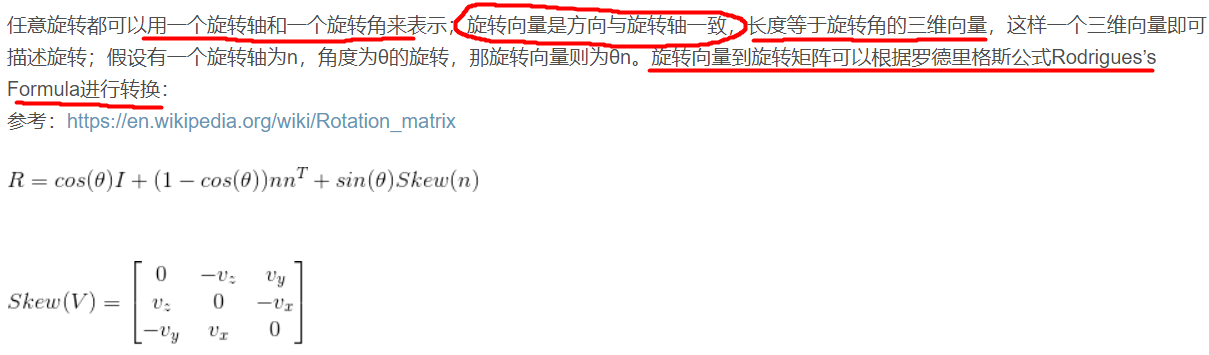R = I3 + torch.sin(theta).view(-1, 1, 1) * Sn \
+ (1. - torch.cos(theta).view(-1, 1, 1)) * torch.matmul(Sn, Sn)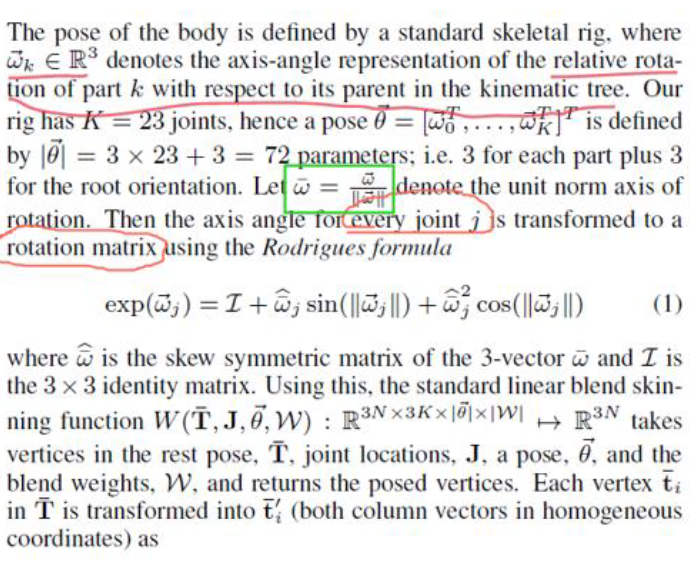def rodrigues(r):
theta = torch.sqrt(torch.sum(torch.pow(r, 2), 1))

def S(n_):
ns = torch.split(n_, 1, 1)
Sn_ = torch.cat([torch.zeros_like(ns), -ns, ns, ns, torch.zeros_like(ns), -ns, -ns, ns,
torch.zeros_like(ns)], 1)
Sn_ = Sn_.view(-1, 3, 3)
return Sn_

n = r / (theta.view(-1, 1))
Sn = S(n)

# R = torch.eye(3).unsqueeze(0) + torch.sin(theta).view(-1, 1, 1)*Sn\
#        +(1.-torch.cos(theta).view(-1, 1, 1)) * torch.matmul(Sn,Sn)

I3 = Variable(torch.eye(3).unsqueeze(0).cuda())

# R等于 公式 (1)---SMPL
R = I3 + torch.sin(theta).view(-1, 1, 1) * Sn \
+ (1. - torch.cos(theta).view(-1, 1, 1)) * torch.matmul(Sn, Sn)

Sr = S(r)
theta2 = theta ** 2
R2 = I3 + (1. - theta2.view(-1, 1, 1) / 6.) * Sr \
+ (.5 - theta2.view(-1, 1, 1) / 24.) * torch.matmul(Sr, Sr)

idx = np.argwhere((theta < 1e-30).data.cpu().numpy())

if (idx.size):
R[idx, :, :] = R2[idx, :, :]

return R, Sn


##### 3.2.3 get_poseweights (poses, bszie)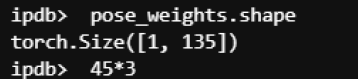• ① 先将 poses([bs, 16, 3])送 入3.2.2所介绍的rodrigues函数中, 按照SMPL2015的说法， 其目的是将每个joint的 axis-angle转为rotation matrix.
pose_matrix为 [15xbs, 3, 3]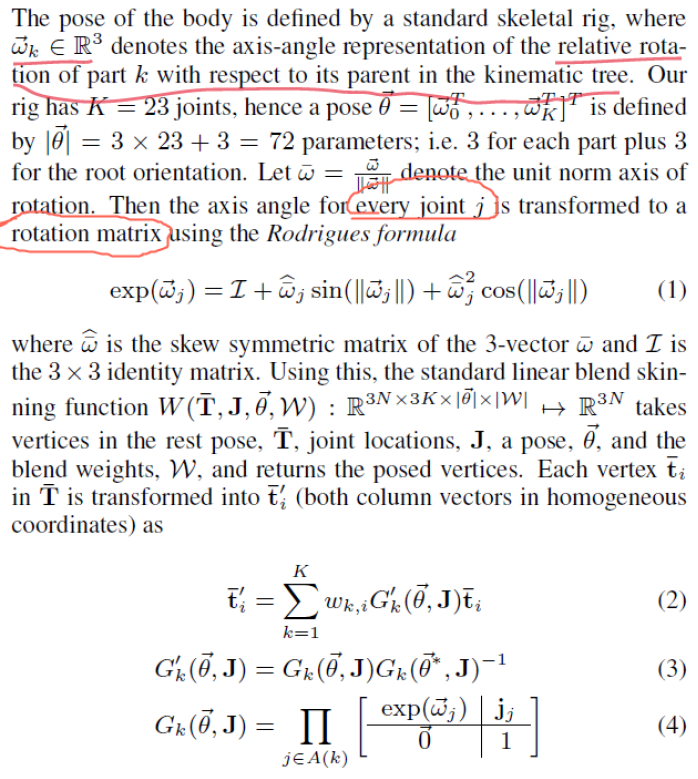• ② pose_matrix 减去单位矩阵, 理解为 (MANO2017)公式 3的 R n ( θ ) − R n ( θ ∗ ) R_n(θ)- R_n(θ^*) :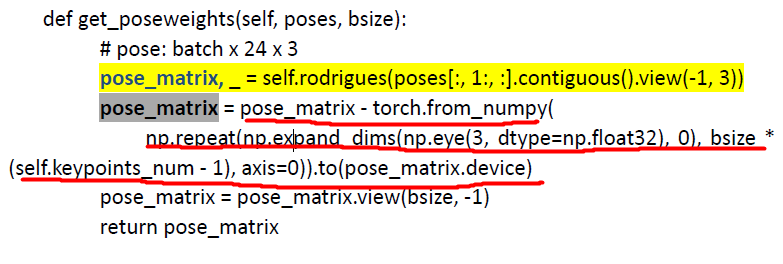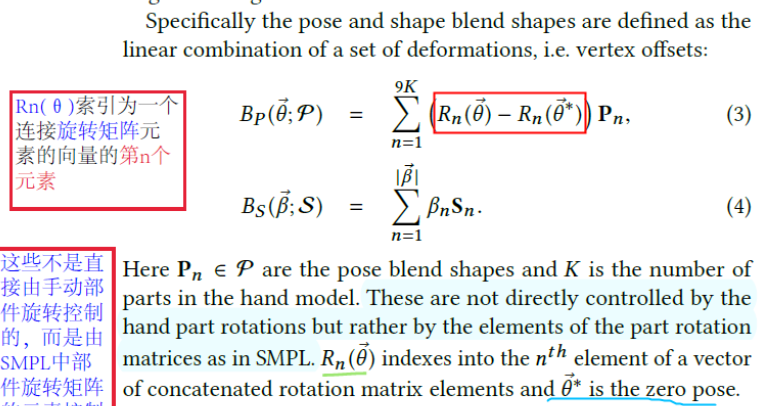def get_poseweights(poses, bsize):
# pose: batch x 24 x 3
pose_matrix, _ = rodrigues(poses[:, 1:, :].contiguous().view(-1, 3))
# pose_matrix, _ = rodrigues(poses.view(-1,3))
pose_matrix = pose_matrix - Variable(torch.from_numpy(
np.repeat(np.expand_dims(np.eye(3, dtype=np.float32), 0), bsize * (keypoints_num - 1), axis=0)).cuda())
pose_matrix = pose_matrix.view(bsize, -1)
return pose_matrix


#### 3.3 rot_pose_beta_to_mesh梳理

① 第一步 是 计算 posesv_shaped.

⚫ poses: poses是网络预测的axis angle + hand_mean (rest pose), 再加上 root_rot(wrist的位置， 代码里面写为 (0,0,0), 是相对位置, 构造root-relative的结果). [bs, 16, 3]

⚫ v_shaped: 对应 SMPL2015的公式10里面的 T ‾ \overline{T} + Bs部分. [bs, 778, 3]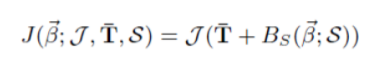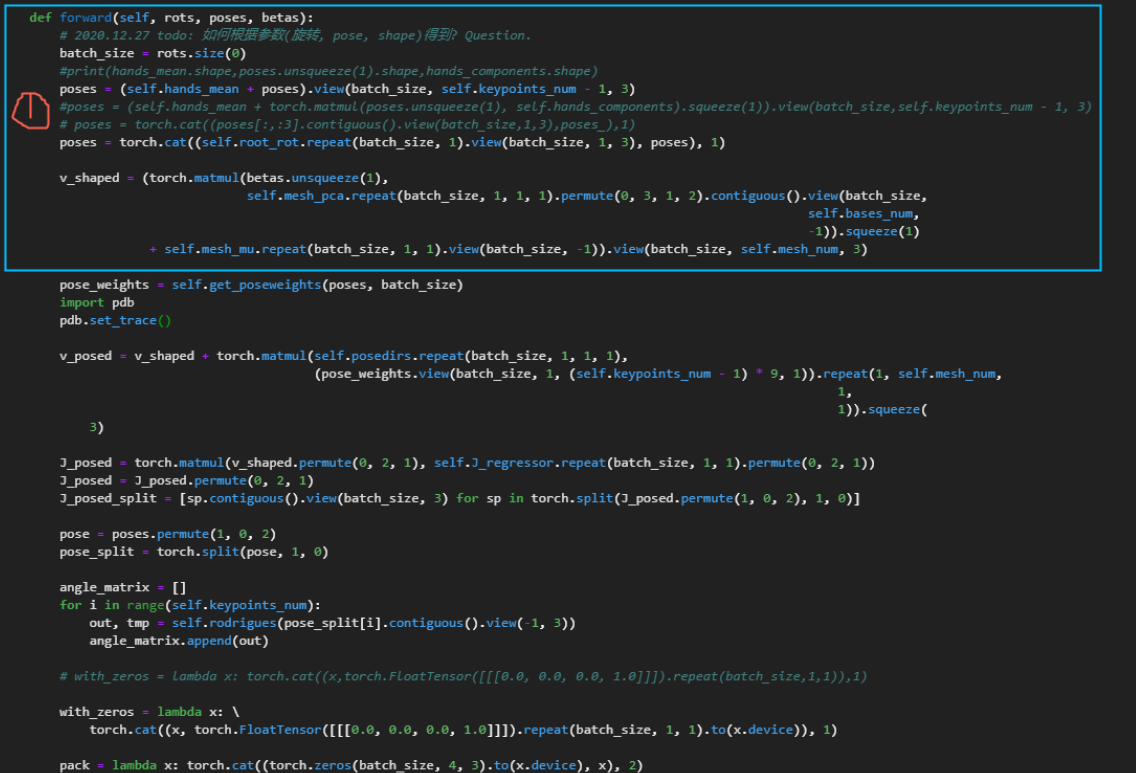② 第 2步 是 计算 pose_weights, v_posedJ_posed.

⚫ pose_weights 是 通过

pose_weights = get_poseweights(poses, batch_size)


⚫ v_posed 是 <3D Hand Shape and Pose from Images in the wild, CVPR2019>的公式 2的 T ( β , θ ) T(β, θ) , 也是 MANO2017的公式 2: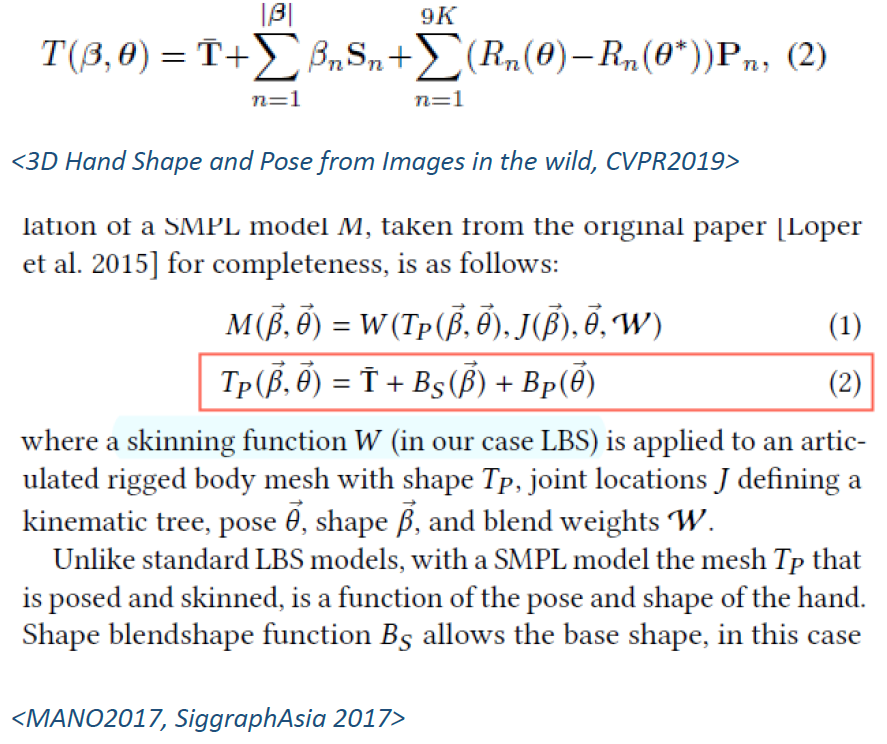⚫ J_posed 是 SMPL2015的 公式 10 其 作用 是 将 vertices变为 joints。([bs, 16, 3])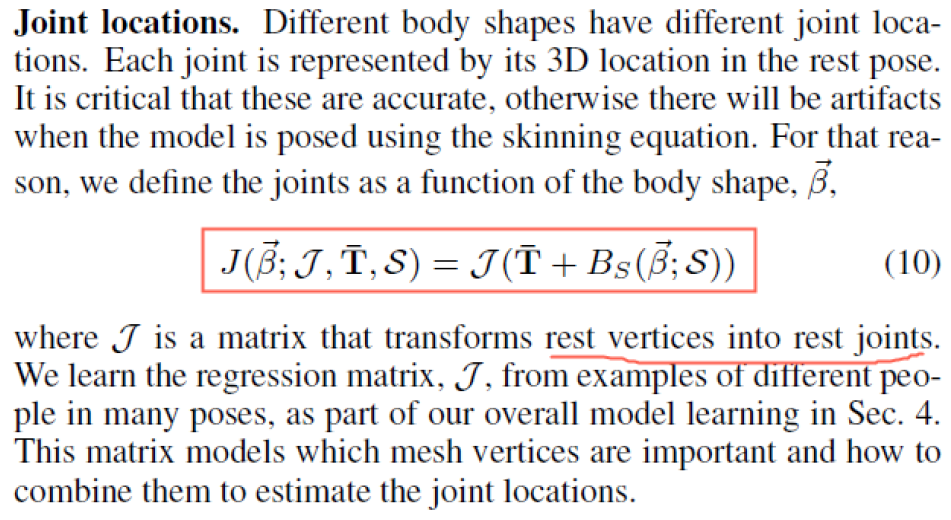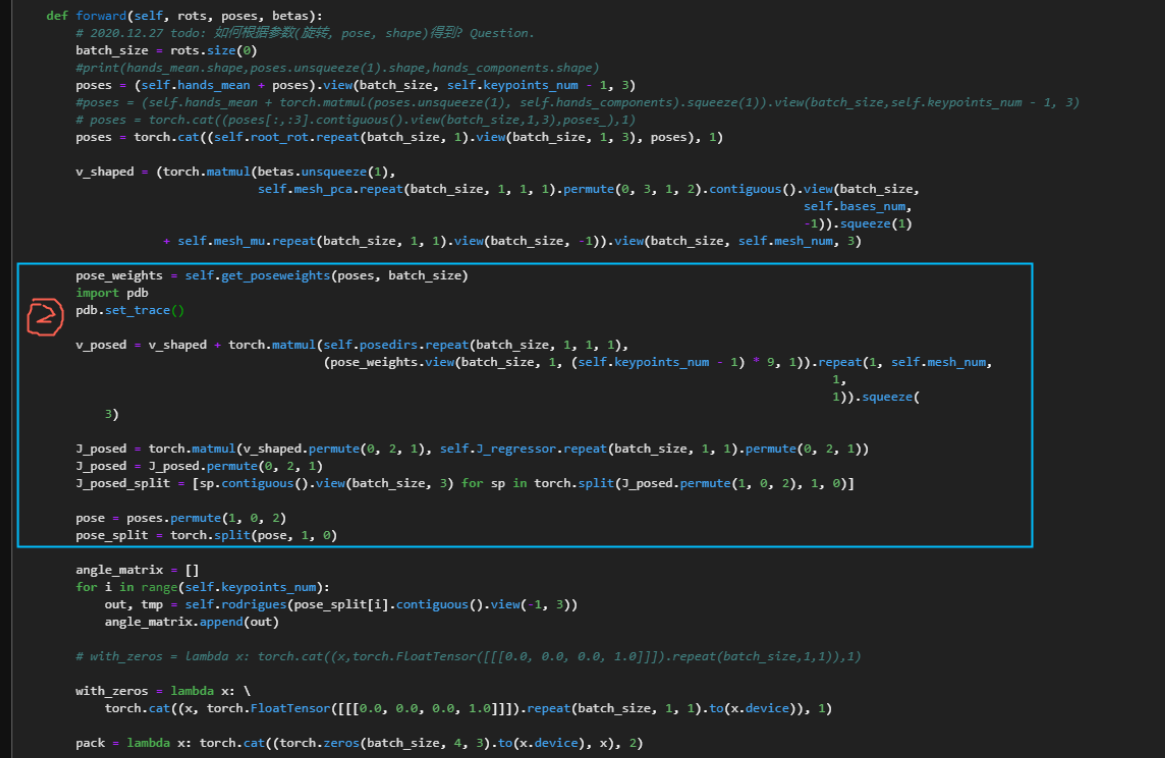③ 第3步是 计算 results_global, 和 T, v.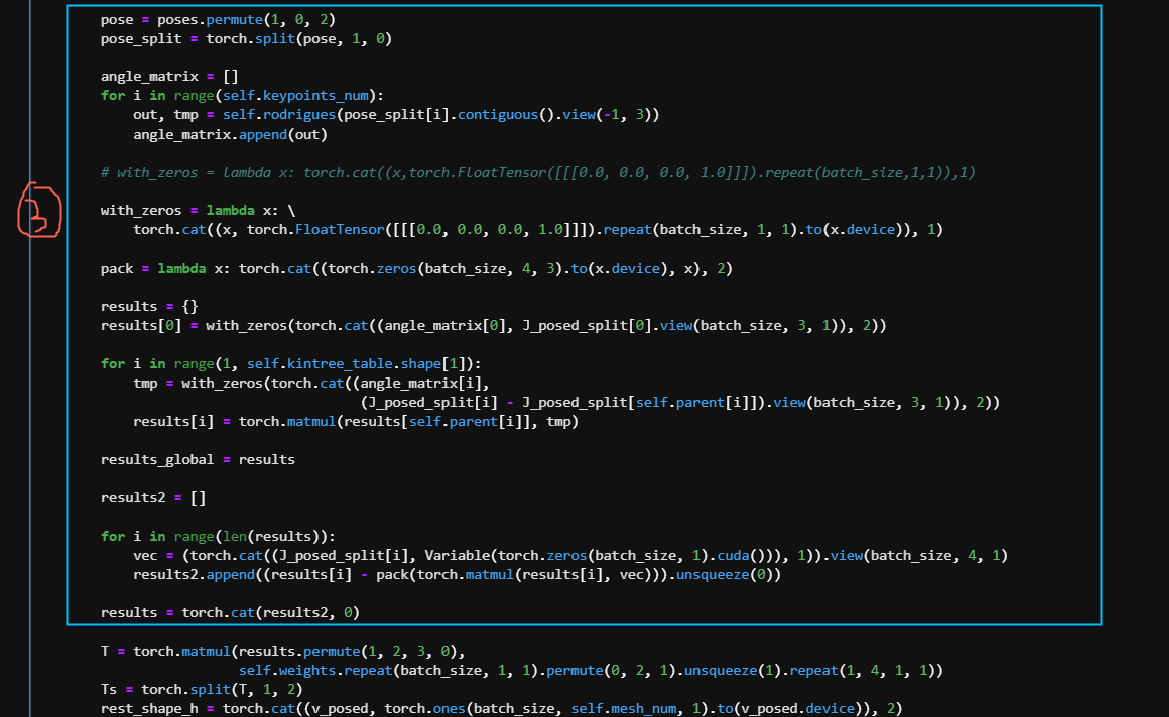⚫ 从J_posed (bs, 16, 3)变为J_posed_split(16, bs, 3). 即这里的处理逻辑是按手指的节点去处理, 而非batch-wise维度的。

⚫ 同理， 有从poses得到的poses_split, 再由poses_split得到angle_matrix.

results_global是根据 kinematic tree来计算的. 每个当前节点的joint location是根据其父节点以及当前节点的旋转矩阵进行矩阵相乘得到的.

results_global也是16个手指节点的 3D xyz. [(bs ,4, 4), (bs, 4, 4), … , (bs ,4, 4)].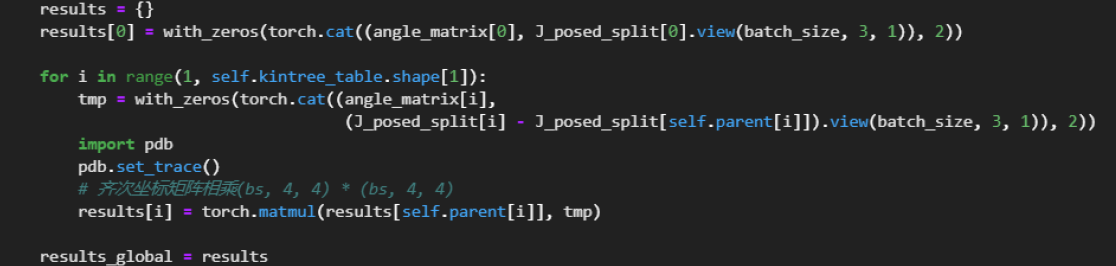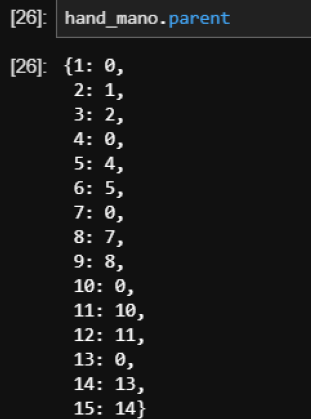T是根据前面的results和weights相乘得到的，这里的 weights是蒙皮blend weights W W .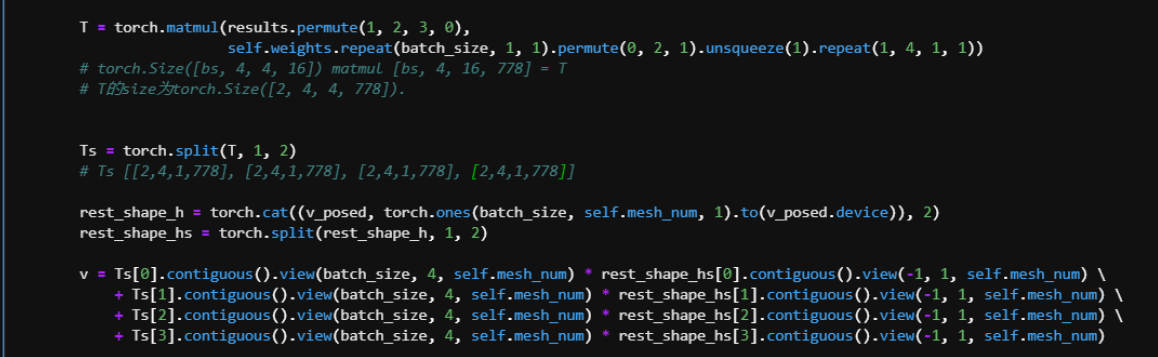V是 经过蒙皮处理后的最终变形节点, Jtr是最终的手部节点 3D位置的集合， 需要注意的是，**指尖(TIP骨骼)**的5个joint的位置是根据 V得到 的.

VJtr是要再旋转 (根据root节点的旋转角)一下输出的才是最终正确结果.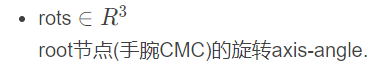④ 最后一步，要从标准的MANO模型里，取TIP骨骼的joint的位置，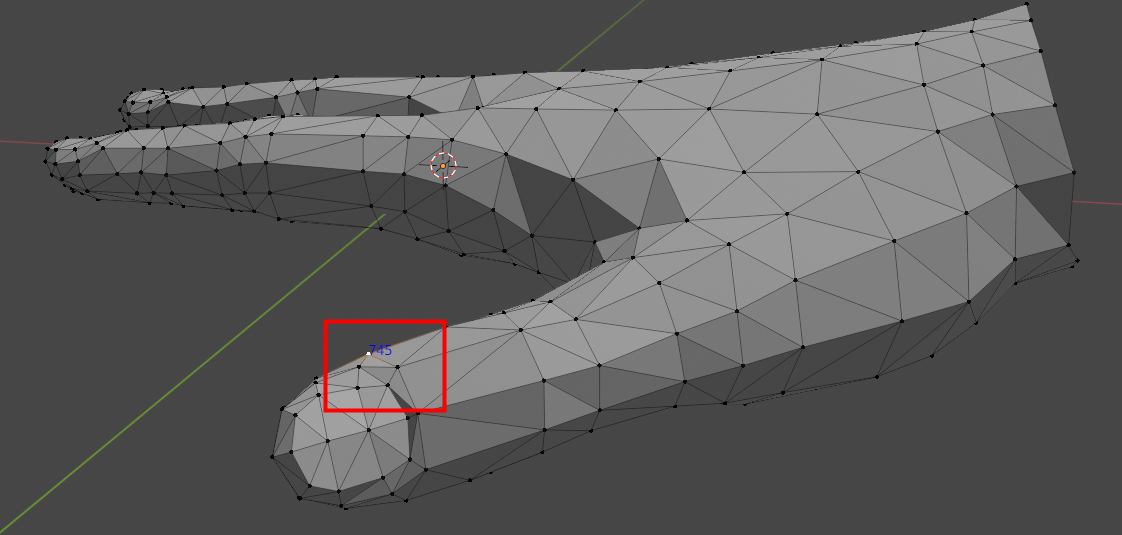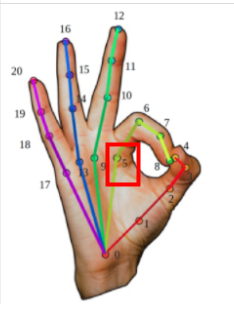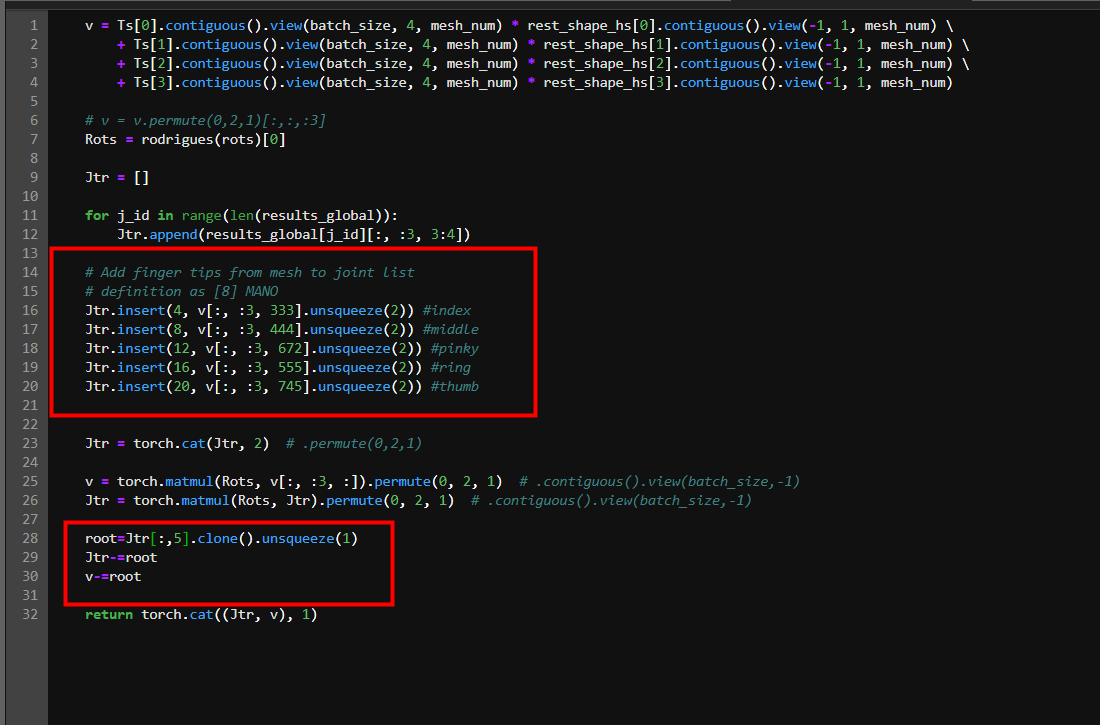### 参考文献

06-23878

05-121279
11-061018
05-11869
03-062万+
03-162303
10-281067
07-151671
12-16624
01-133134
10-27985
04-01
01-062914
03-05
01-12184
04-192768
01-021765
04-26

### “相关推荐”对你有帮助么？

•非常没帮助
•没帮助
•一般
•有帮助
•非常有帮助

©️2022 CSDN 皮肤主题：Age of Ai 设计师：meimeielliesooner高

¥2 ¥4 ¥6 ¥10 ¥20余额支付 (余额：-- )扫码支付获取中扫码支付点击重新获取扫码支付1.余额是钱包充值的虚拟货币，按照1:1的比例进行支付金额的抵扣。
2.余额无法直接购买下载，可以购买VIP、C币套餐、付费专栏及课程。余额充值